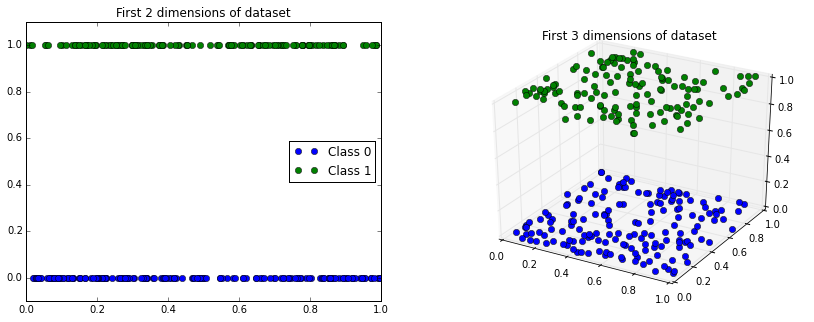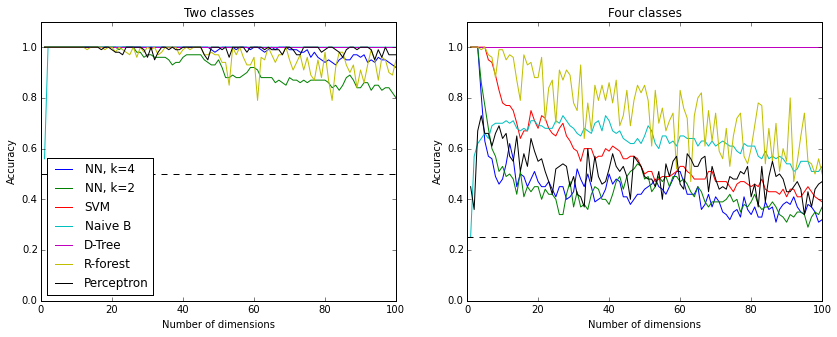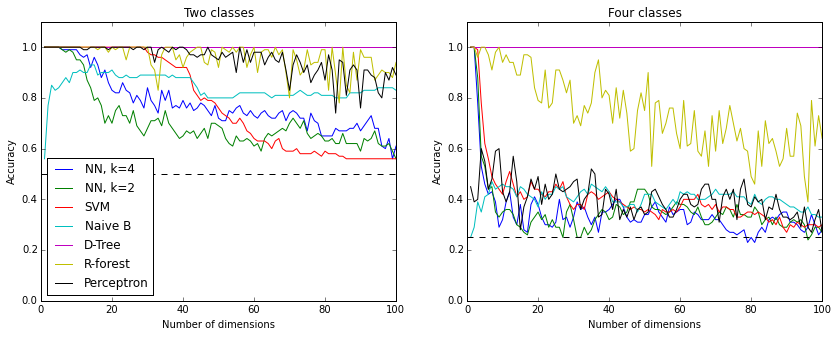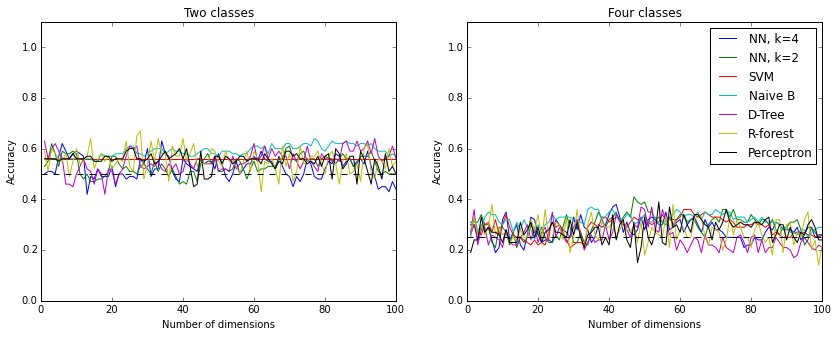# Algorithms sensitivity to single salient dimension

Posted on Fri 23 January 2015 in Notebooks

# Sensitivity to 1 salient dimension¶

## How different classifiers managers to sort through noise in multidimensional data¶

In this experiment I will test different machine learning algorithms sensitivity to data where only 1 dimension is salient and the rest are pure noise. The experiment tests variations of saliency against a number of dimensions of random noise to see which algorithms are good at sorting out noise.
For experiments performed here, there will be a 1-1 mapping between the target class in $y$ and the value of the first dimension in a datapoint in $x$. For example, for all datapoints belonging to class 1, the first dimension will have the value 1, while if the datapoint belongs to class 0, the first dimension will have the value 0.

In :
#Configure matplotlib
import matplotlib.pyplot as plt
from mpl_toolkits.mplot3d import Axes3D
%pylab

#comment out this line to produce plots in a seperate window
%matplotlib inline
figsize(14,5)

Using matplotlib backend: MacOSX
Populating the interactive namespace from numpy and matplotlib


# Data¶

First the target vectors $y$ and $y_4$ are randomly populated. $y\in[0,1]$ and $y_4\in[0,1,2,3]$. The for each value in $y$ and $y_4$ a datapoint is generated consisting of the value of the target class, followed by 100 random values. This way the first column in the data matrix is equal to the target vector. Later this column will be manipulated linearly.

In :
#initialize data

def generate_data():
''' Populates data matrices and target vectors with data and release it into the global namespace
running this function will reset the values of x, y, x4, y4 and r '''
global x, y, x4, y4, r
y = np.random.randint(2, size=300)
y4 = np.random.randint(4, size=300)
r = np.random.rand(100, 300)
x = np.vstack((y,r)).T
x4 = np.vstack((y4,r*4)).T

generate_data()

# note that x and y are global variables. If you manipulate them, the latest manipulation of x and y will
# used to generate plots.

split = 200
max_dim = x.shape
m = 1
y_cor = range(m, max_dim)

print 'y is equal to 1st column of x:  \t', list(y) == list(x[:,0])
print 'y4 is equal to 1st column of x4:\t', list(y4) == list(x4[:,0])
print '\nChecking that none of the randomized data match the class values
print 'min:\t', r.min(), 'max:\t',r.max(), '\tThese should never be [0,1], if so please rerun.'

y is equal to 1st column of x:  	True
y4 is equal to 1st column of x4:	True
min:	5.75157395193e-05 max:	0.999952558364 	These should never be [0,1]


## Visualizing the data¶

This section will plot parts of the data to give the reader a better understanding of its shape.

In :
plt.subplot(121)
plt.title('First 2 dimensions of dataset')
plt.plot(x[np.where(y==0)][:,1],x[np.where(y==0)][:,0], 'o', label='Class 0')
plt.plot(x[np.where(y==1)][:,1],x[np.where(y==1)][:,0], 'o', label='Class 1')
plt.ylim(-0.1, 1.1) #expand y-axis for better viewing
legend(loc=5)

plt.subplot(122, projection='3d')
plt.title('First 3 dimensions of dataset')
plt.plot(x[np.where(y==0)][:,2],x[np.where(y==0)][:,1],x[np.where(y==0)][:,0], 'o', label='Class 0')
plt.plot(x[np.where(y==1)][:,2],x[np.where(y==1)][:,1],x[np.where(y==1)][:,0], 'o', label='Class 1')

Out:A clear seperation between classes is revealed when visualized. This clear seperation between the 2 classes remains, no matter how many noisy dimensions we add to the dataset, so in theory it is reasonable to expect any linear classifier to find a line that seperates the 2 datasets.

In :
#Initialize classifiers

from sklearn.neighbors import KNeighborsClassifier as NN
from sklearn.svm import SVC as SVM
from sklearn.naive_bayes import MultinomialNB as NB
from sklearn.lda import LDA
from sklearn.tree import DecisionTreeClassifier as DT
from sklearn.ensemble import RandomForestClassifier as RF
from sklearn.linear_model import Perceptron as PT

classifiers = [NN(),NN(n_neighbors=2), SVM(), NB(), DT(), RF(), PT()]
titles = ['NN, k=4', 'NN, k=2', 'SVM', 'Naive B', 'D-Tree', 'R-forest', 'Perceptron']

# uncomment the following to add LDA
#classifiers = [NN(),NN(n_neighbors=2), SVM(), NB(), DT(), RF(), PT(), LDA()]
#titles = ['NN, k=4', 'NN, k=2', 'SVM', 'Naive B', 'Perceptron', 'LDA']
#m, y_cor= 2, range(m, max_dim)

In :
# define functions
def run (x,y):
'''Runs the main experiment. Test each classifier against varying dimensional sizes of a given dataset'''

global score
score = []
for i, clf in enumerate(classifiers):
score.append([])
for j in range(m,max_dim):
clf.fit(x[:split,:j],y[:split])
score[i].append(clf.score(x[split:,:j], y[split:]))

def do_plot():
''' Generates the basic plot of results
Note:   Score is a global variable. The latest score calculated from run()
will always be used to draw a plot '''

for i, label in enumerate(titles):
plt.plot(y_cor, score[i], label=label)
plt.ylim(0,1.1)
plt.ylabel('Accuracy')
plt.xlabel('Number of dimensions')

def double_plot():
''' Runs the experiment for 2 classes and 4 classes and draws appropriate plots
Note:   x and y are global variables. The latest manipulation of these are
always used to run the experiment. If you need 'original' x and y's
you need to rerun generate_data() and use new randomized data '''

plt.subplot(121)
plt.title('Two classes')
run(x,y)
do_plot()
plt.legend(loc=3)
plt.subplot(122)
plt.title('Four classes')
run(x4,y4)
do_plot()


# Experiment 1¶

Test all classifiers against 2 class and 4 class datasets for 1 through 100 dimensions. Notice that Naive Base fails when the dataset is literally equal to the targets, but adding just a little bit of noise and it starts to work much better.

For 4 classes, Naive Base again starts of poorly, but while the other algorithms quickly succumb to the noisy dimensions, Naive Bayes seems to improve up until ~20 dimension, and though its performs starts to decline, it is still the best performer from there on out. If you are running the experiment with LDA, then the test will not be done for 1 dimension, and Naive Bayes weakest point won't show.

However, the Decision Tree is quick to find that one dimension explains everything, and has no trouble throughout either experiment. The random forest have some trees where the salient dimension has been cut off, so more noise and randomness is added to the results.

In :
double_plot()# Experiment 2¶

In this experiment the first column of the data matrix is linearly manipulated in order to "hide" the values that map to the classes better amongst the noise. For 2 classes experiment the value 0.25 maps to class 0 and 0.75 maps to class 1. For the 4 class experiment, value:class mapping is now 1:0, 1.5:1, 2:2, 2.5:3, 3:4 This does not change the fact that there is a clear boundry between the classes. It just means the distance between the 2 planes seen in the visualization section is getting narrower.

In :
plt.figure()
x[:,0] = (x[:,0]/2)+0.25
x4[:,0] = (x4[:,0]/2)+1

double_plot()This experiment is quite sensitive to the randomness in the data. For two classes in general the SVM is the strongest until around the 40 dimension mark, where the Naive Bayes takes over. In the higher dimension area, NN often manages to overtake SVM, though this is somewhat dependent on the random data. It's still surprising, given that NN is usually the poster child for the curse of dimensionality. It is not that easy to hide linear explanation for to the Decision Tree, which clearly outperforms everything here. The tendency to overfitting is really helping.

# Baseline test (random only)¶

In this final experiment the only salient datapoint in the observation data is removed to show the reader that this will attain baseline results. Also notice, depending on the data, the baseline for some of the algorithms can be as high as 60% accuracy. Keep this in mind when reviewing results from above.

In :
x = x[:,1:]
x4 = x4[:,1:]

double_plot()
plt.legend(loc=1)
plt.subplot(121)
plt.legend().remove()